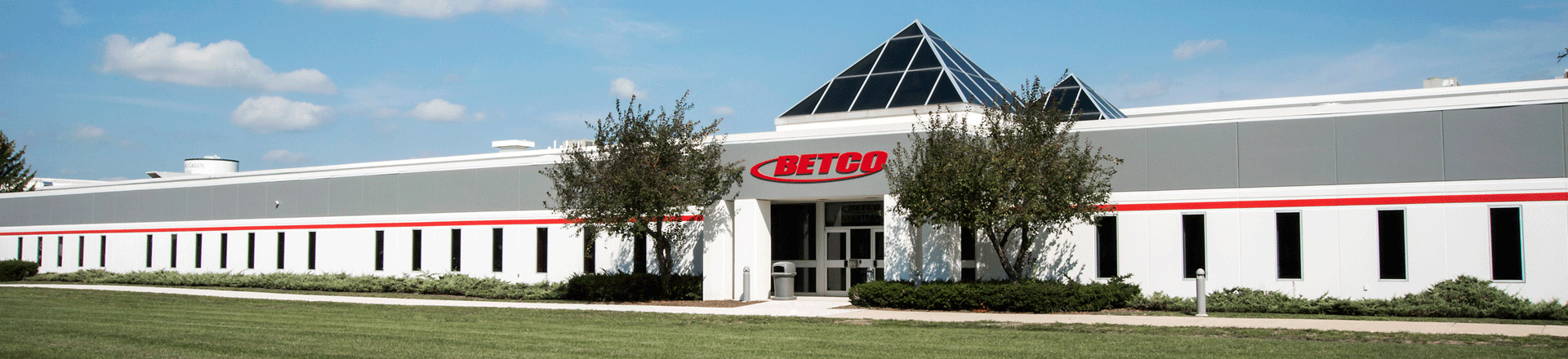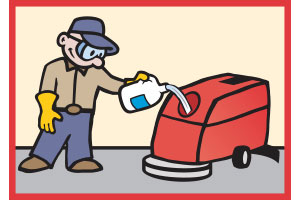Blog

Home > MyBetco > Blog

## A Quick Guide to Calculating Dilution Ratios

Sep 24, 2018Let’s face it – calculating dilution ratios can be complicated.

Since cleaning products can have different dilution ratios, it’s important to understand how to properly dilute the product. Here’s a quick guide to help get you there.

Starting with the Basics

Always remember that there are 128 ounces in one gallon. This is important to know when working with dilution ratios.

Dilution ratios are expressed in two ways:

1. Typically, you’ll see dilution ratios expressed as 1 to a given number such as 1:256.

If a dilution ratio is expressed in this way, you will have to calculate the ounces per gallon.

A common method to determine ounces per gallon is to take 128 (because that’s how many ounces are in a gallon) and divide it by the ratio number. Like this:

128 ÷ ratio number = ounces per gallon

Now let’s plug in some numbers! For example, a product that has a dilution ratio of 1:256 would be calculated like this:

128 ÷ 256 = .5 ounces per gallon

In this example, 256 is our ratio number and by plugging it into our formula, we can determine how many ounces per gallon of product is needed.

2. Dilution ratios can also be expressed in terms of ounces per gallon such as .5 ounces per gallon – no math needed here!

Both ways mean the same thing – you would mix one part of the product to 256 parts water. This means that the gallon of product will make 257 total gallons of solution because to mix the proper solution, you would take the gallon of product and add 256 gallons of water which equals 257 total gallons.Dilution Ratio Cheat Sheet

Take the guesswork out of it! To help keep things simple, here are some common dilution ratios:

 Ounces per Gallon Dilution Ratios ¼ ounce per gallon 1:512 ½ ounce per gallon 1:256 1 ounce per gallon 1:128 2 ounces per gallon 1:64 4 ounces per gallon 1:32 5 ounces per gallon 1:26 (1:25.6 rounded) 6 ounces per gallon 1:21 (commonly considered 1:20) 8 ounces per gallon 1:16 12 ounces per gallon 1:10

Need a Metric Calculation?

Metric calculations such as liters can also be calculated. You just need to convert the metric figures to gallons or ounces prior to starting. Common metric volume measurements and their English equivalents are listed below:

1 quart = 0.946 mL
1 gallon = 3.785 liters
1 liter = 0.264 gallons
4 liters = 1.056 gallonsThe FastDraw® System

The Betco® FastDraw® chemical management system consistently provides accurate dilution every time with innovative, versatile, and simple systems that use a one size fits all FastDraw bottle. With many types available, there is bound to be a system that meets the needs of your facility.

• FastDraw 1 is a wall mounted system with the capability to dilute multiple products from a single dispenser. It is most ideal for a small or large cleaning that requires a single versatile dispenser.
• FastDraw PRO® is a multi-product dispensing system that is able to hold 4 concentrates to quickly dispense from a flat wall or in a corner. It is most ideal for areas with multiple cleaning requirements.
• FastPak™ takes dilution control on the go by providing precise dosing anywhere. Eliminate overdosing and save on concentrates by measuring ¼ to 3 oz. accurately every time.

When cleaning products are not accurately diluted, it can result in a waste of labor, cost, and poor performance. By consistently diluting the correct amount of chemical when filling spray bottles, mop buckets, and automatic scrubbers, cleaning costs are reduced by 30%.